Computational & Technology Resources
an online resource for computational,
engineering & technology publications
Civil-Comp Proceedings
ISSN 1759-3433
CCP: 84
PROCEEDINGS OF THE FIFTH INTERNATIONAL CONFERENCE ON ENGINEERING COMPUTATIONAL TECHNOLOGY
Edited by: B.H.V. Topping, G. Montero and R. Montenegro
Paper 192

Non-Linear Field Computation with Hysteresis

A. Schiffer and A. Iványi

Department of Information Technology, Pollack Mihály Faculty of Engineering, University of Pécs, Hungary

Full Bibliographic Reference for this paper
A. Schiffer, A. Iványi, "Non-Linear Field Computation with Hysteresis", in B.H.V. Topping, G. Montero, R. Montenegro, (Editors), "Proceedings of the Fifth International Conference on Engineering Computational Technology", Civil-Comp Press, Stirlingshire, UK, Paper 192, 2006. doi:10.4203/ccp.84.192
Keywords: finite element modelling, hysteresis, magnetic force.

Summary
In this paper a one-dimensional computational problem has been introduced with nonlinear hysteresis. The materials have been modeled with the well-known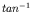hysteresis characteristic between the magnetic field intensity H and the magnetization M. In the paper a finite element (FE) method is combined with modeling of ferromagnetic material. Post processing of the nonlinear field problem yields the investigation of magnetic forces between the plates. The final purpose is to study the energy losses and the vibration generated sound due to the magnetic forces.

The goal of this paper is to derive the magnetic field intensity H inside two infinite large plates with d=7mm and compute the magnetic forces between the plates.

The governing equations of the FE model can be described by Maxwell's equations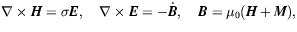(97)

and the following equation yielding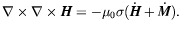(98)

Reducing for the one-dimensional problem in direction z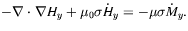(99)

For the nonlinear material modeling the hysteresis characteristic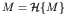withfunction is described as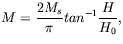(100)

where H0 the magnetic field intensity at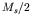and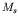is the value of the magnetization in saturation. For linear material modeling the relationship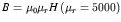has been used.

The model has been applied to a one-dimension problem in the z direction with sinusoidal excitation at the boundaries. The Dirichlet boundary conditions are defined as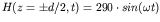with an excitation frequency of f=500Hz.

The magnetic force along z direction can be calculated by the following equation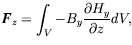(101)

where By is the variation of the magnetic flux density induced in the material by the magnetic field along the z direction. Different numerical techniques have been considered for the time step process. The solution is calculated by combining a Comsol FE solver for the time independent, stationary nonlinear problem and a fixed-point iteration for every time step. In Figure 1 the H field deformation due to the characteristic between H and M can be seen, where different H0 values occurs different diffusion behavior.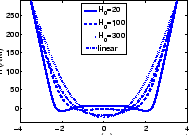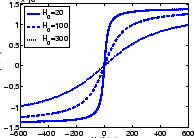purchase the full-text of this paper (price £20)